# ECMAScript Arrays Tutorial

## 1- What is Array ?

In  ECMAScript, an array is a collection of consecutive positions in memory to store data. Each memory position is called an element.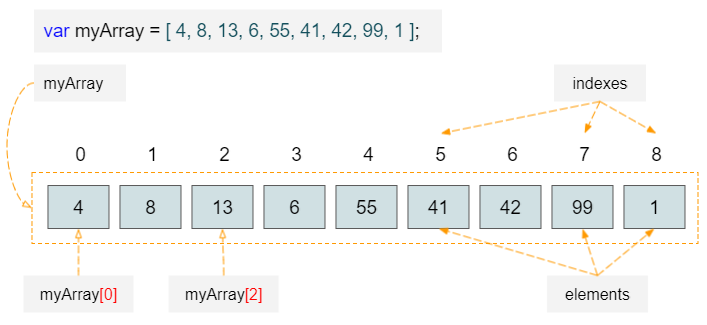Below are array characteristics:
• Elements are indexed (0, 1, 2,..) starting by 0, and you can can access an element by its index.
• Arrays are also like variables. You must declare it before using it.
• You can update or edit the value of an element in an array.

### Why is an array necessary?

Instead of declaring an array with N elements, you can declare N variables, but it has the following weakpoints:
1. If N is a large number, it is not feasible to declare N variables manually
2. When you declare N variables, the program doesn't manage the order of variables for you because there is no concept of index for variables.

## 2- Declare array

Like variables, you have to declare an array before using it.
```// Declaration
var myArray;

// Initialization
myArray = [val1, val2, .., valN]

// Or

var myArray = [val1,val2, .., valN]
```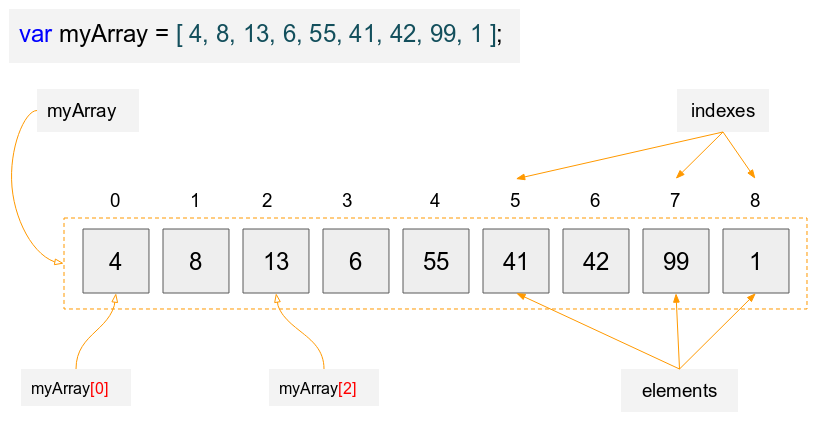array-example1.js
```
var myArray = [ 4, 8, 13, 6, 55, 41, 42, 99, 1 ];

console.log("Length of myArray: " + myArray.length); // 9 Elements

console.log("Element at index 0: " + myArray); // 4

console.log("Element at index 1: " + myArray); // 8

console.log("Element at index 4: " + myArray); // 55
```
You can assign a new value to an array element
array-example2.js
```
var names = [ "Trump", "Putin", 100 ];

console.log("Length of array: " + names.length); // 3 Elements

console.log("Element at index 0: " + names); // Trump

console.log("Element at index 1: " + names); // Putin

console.log("Element at index 2: " + names); // 100

// Assign new value to element at index 2:
names = "Merkel";

console.log("Element at index 2: " + names);
```
Using loops, you can access array elements respectively:
array-loop-example.js
```
var fruits = ["Acerola", "Apple", "Banana", "Breadfruit", "Carambola" ];

for( var index = 0; index < fruits.length; index++) {
console.log("Index: " + index+" Element value: " + fruits[index]);
}
```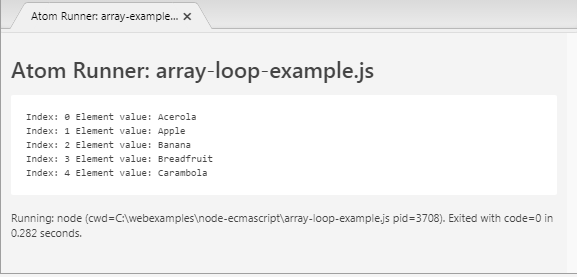## 3- Array Object

An array can also be created via the  Array class:
```
// Create an array has 4 elements
var myArray1 = new Array(4);

// Create an array with 3 elements:
var myArray2 = new Array("Acerola", "Apple", "Banana");
```
Example:
array-object-example.js
```
// Create an array has 4 elements:
var salaries = new Array(4);

for(var i = 0; i < salaries.length; i++) {

console.log(salaries[i]); // undefined
}

for(var i = 0; i < salaries.length; i++) {

// Assign new value to element:
salaries[i] = 1000 + 20 * i;
}

console.log(salaries); // 1040.
```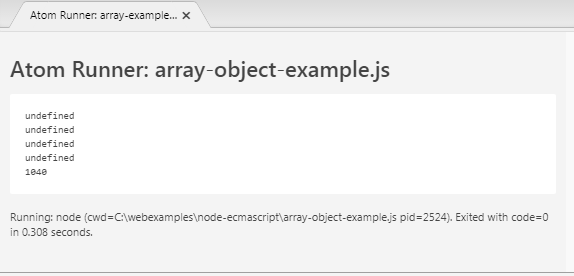## 4- Array methods

An array is an object. It will have methods. Below is the list of its methods:
• concat()
• every()
• some()
• filter()
• forEach()
• map()
• indexOf()
• join()
• lastIndexOf()
• pop()
• push()
• reduce()
• reduceRight()
• reverse()
• shift()
• slice()
• toSource()
• sort()
• splice()
• toString()
• unshift()

### concat()

The  concat() method returns a new array including its elements joining with the elements of arrays (or values) in parameters .
```// Example:

array.concat(arrayOrValue1, arrayOrValue2, ..., arrayOrValueN);
```
Parameters:
• arrayOrValueN: An array or a value.
array-concat-example.js
```
var alpha = ["a", "b", "c"];

var numeric = [1, 2, 3];

var results = alpha.concat(numeric, "!");

console.log(alpha); // ["a", "b", "c"];

console.log(results); // [ 'a', 'b', 'c', 1, 2, 3, '!' ]

```

### every()

This method returns  true if all elements of this aray satisfy a test function provided .
```array.every(callback[, thisObject]);
```
Parameters:
• callback − Function to test for each element.
• thisObject − Object to use as this when executing callback.
For example, you have an array of values of employees' ages, let's use the  every() method to test whether all elements of the array are located within 18-60 or not.
array-every-example.js
```
// Test if value between 18 and 60.
testAge = function(value, index, testArray)  {

return value >= 18 && value <= 60;
}
var ages = [18, 22, 59];

// Or: var okAll = ages.every(testAge);
var okAll = ages.every(testAge, ages);

console.log("OK ALL? " + okAll);
```

### some()

Returns true if at least one element in this array satisfies the provided testing function.
```array.some(callback[, thisObject]);

```
Parameters:
• callback − Function to test for each element.
• thisObject − Object to use as this when executing callback.
array-some-example.js
```
// Test if value between 18 and 60.
testAge = function(value, index, testArray)  {

return value >= 18 && value <= 60;
}
var ages = [15, 17, 22, 80];

// Or: var ok = ages.some(testAge);
var ok = ages.some(testAge, ages);

console.log("OK? " + ok); // true
```

### filter()

This method creates a new array including its elements which pass the test of a function provided.
```array.filter(callback[, thisObject]);
```
Parameters:
• callback − A Function to test for each element. Returns true/false.
• thisObject − Object to use as this when executing callback.
Example:
array-filter-example.js
```
// Test if value between 18 and 60.
// (callback function)
testAge = function(value, index, testArray)  {

return value >= 18 && value <= 60;
}
var ages = [5, 6, 18, 22, 59, 15 ];

// Or: var validAges = ages.filter(testAge);
var validAges = ages.filter(testAge, ages);

console.log("Valid Ages: ");
console.log(validAges); // [ 18, 22, 59 ]
```

### forEach()

This method will call a function for each element of array.
```array.forEach(callback[, thisObject]);
```
Parameters:
• callback − A function to do something for each element.
• thisObject − Object to use as this when executing callback.
array-forEach-example.js
```
// Check value between 18 and 60.
// (callback function)
showTestResult = function(value, index, testArray)  {
if(value >= 18 && value <= 60) {
console.log("Age " + value + " valid!");
} else  {
console.log("Sorry, Age " + value + " invalid!");
}
}
var ages = [5, 6, 18, 22, 59, 15 ];

// Or: ages.filter(showTestResult);
ages.forEach(showTestResult, ages);

```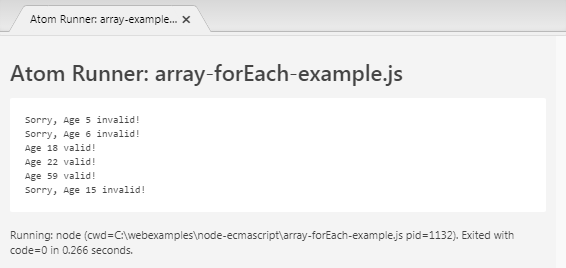### map()

This method returns a new array with the number of elements which is equal to the number of the elements of this array. By calling a function for each element of this array to calculate the value for the corresponding element of the new array.
```
array.map(callback[, thisObject]);

```
Parameters:
• callback − The function that is used to create an element of new Array from an element of the current one.
• thisObject − Object that is used as this when executing the callback function.
A simple example: Suppose that you have an array of elements, simulating staff salaries and you want to calculate an array of personal income tax for staff, the rules are:
• If salary > 1000, tax is 30%.
• If salary > 500, tax is 15%.
• In contrast, tax is 0%.
array-map-example.js
```
var taxFunc = function(value, index, thisArray)  {
if(value > 1000)  {
return value * 30 / 100;
}
if( value > 500)  {
return value * 15 / 100;
}
return 0;
}

var salaries = [1200, 1100, 300, 8000, 700, 200 ];

//
var taxes = salaries.map(taxFunc, salaries);

console.log(taxes); // [ 360, 330, 0, 2400, 105, 0 ]
```
The   Math.abs(x) function returns some "absolute value" of  x. This is the function built in  Javascript.
array-map-example2.js
```var array1 = [-3, 5, -10];

//
var array2 = array1.map(Math.abs, array1);

console.log(array2); // [ 3, 5, 10 ]
```

### indexOf()

Returns the first (least) index of an element within the array equal to the specified value, or -1 if none is found.
```
array.indexOf(searchValue [, fromIndex]);

```
Parameters:
• searchValue − Value to search in the array.
• fromIndex −The index at which to begin the search. Defaults to 0.
array-indexOf-example.js
```//             0   1   2   3   4   5
var array1 = [10, 20, 30, 10, 40, 50];

var idx = array1.indexOf(10, 1);

console.log(idx); // 3
```

### lastIndexOf()

Returns the last (greatest) index of an element within the array equal to the specified value, or -1 if none is found.
```
array.lastIndexOf(searchElement[, toIndex]);
```
Parameters:
• searchValue − Value to search in the array.
• toIndex − The index is searched from 0 to toIndex, default toIndex is equal to the length of the array.
array-lastIndexOf-example.js
```//             0   1   2   3   4   5   6
var array1 = [10, 20, 30, 10, 40, 10, 50];

var idx = array1.lastIndexOf(10, 4);

console.log(idx); // 3
```

### pop()

Removes the last element from an array and returns that element.
```
array.pop();

```
array-pop-example.js
```
var fruits = ["Apple", "Banana", "Papaya"];

console.log(fruits); // [ 'Apple', 'Banana', 'Papaya' ]

var last = fruits.pop();

console.log(last); // Papaya

console.log(fruits); // [ 'Apple', 'Banana' ]
```

### push()

This method appends additional new elements to the end of present array and returns the length of array after appending.
```
array.push( value1, ..., valueN);

```
array-push-example.js
```
var fruits = ["Apple", "Banana", "Papaya"];

console.log(fruits); // [ 'Apple', 'Banana', 'Papaya' ]

console.log(newLength); // 5

console.log(fruits); // [ 'Apple', 'Banana', 'Papaya', 'Apricots', 'Avocado' ]
```

### reduce()

This method is used to make calculations on an array and returns a single value.
```
array.reduce(callback [, initialValue]);

```

Parameters:

• callback − This function will be called for each element of array (from left to right). It has two parameters (value, element) and returns a value. Such returned value will be passed to the value parameter for next call.
• initialValue − Value for the value parameter in the first time of the callback funtion.
array-reduce-example.js
```var sum = function(a, b)  {
return a + b;
}

var numbers = [1, 2, 4 ];

//
var total = numbers.reduce(sum, 0);

console.log(total); // 7
```

### reduceRight()

This method is used to make calculations on an array and returns a sole value.
```
array.reduceRight(callback [, initialValue]);

```

Parameters:

• callback − This function will be called for each array element (from right to left). It has two parameters (value, element) and returns a value. Such returned value will be passed to the value parameter for next call.
• initialValue − Value for the value parameter for the first call of the callback function.
array-reductRight-example.js
```var sum = function(a, b)  {
return a + b;
}

var numbers = [1, 2, 4 ];

//
var total = numbers.reduce(sum, 0);

console.log(total); // 7
```

### reverse()

Reverses the order of the elements of an array -- the first becomes the last, and the last becomes the first, ...
array-reverse-example.js
```var numbers = [1, 2, 3, 4 ];

//
numbers.reverse( );

console.log(numbers); // [ 4, 3, 2, 1 ]
```

### shift()

This method eliminates the first element from the array and returns this element.
array-shift-example.js
```
var fruits = ["Apple", "Banana", "Papaya"];

console.log(fruits); // [ 'Apple', 'Banana', 'Papaya' ]

var last = fruits.shift();

console.log(last); // Apple

console.log(fruits); // [ 'Banana', 'Papaya' ]
```

### slice()

This method extracts a section of an array and returns a new array.
```
array.slice( begin [,end] );

```
Parameters:
• begin - beginning index. If this value is negative, it is the same as begin = length - begin (length means array length).
• end - ending index. If this value is negative, it is the same as end = length - end (length means array length).
array-slice-example.js
```
var fruits = ['Apple', 'Banana', 'Papaya', 'Apricots', 'Avocado']

var subFruits = fruits.slice(1, 3);

console.log(fruits); // [ 'Apple', 'Banana', 'Papaya', 'Apricots', 'Avocado' ]

console.log(subFruits); // [ 'Banana', 'Papaya' ]
```

### sort()

#### View more Tutorials:

Maybe you are interested

These are online courses outside the o7planning website that we introduced, which may include free or discounted courses.

•VueJS V1 Introduction to VueJS JavaScript Framework
•JavaScript projects Welcome to the DOM Useful Code Snippets
•JavaScript Fundamentals: A Course for Absolute Beginners
•Learn JavaScript From Scratch:Become Top Rated Web Developer
•Learn Javascript ECMAscript ES6, ES7, ES8, and Beyond Course
•Essentials of JavaScript Practice Coding Exercises Tips
•Build a Real Time Chat App with JavaScript and Firebase
•JavaScript - Start Developing Applications in 2 Hours Free!
•HTML5, CSS3 And JavaScript Fundamentals 2016
•Quick JavaScript Core learning Course JavaScript Essentials
•The Complete JavaScript Course - Beginner to Professional
•Byte-Sized-Chunks: Dynamic Prototypes in Javascript
•The Complete JavaScript Bootcamp
•1 Hour JavaScript
•Getting really good at JavaScript and TypeScript